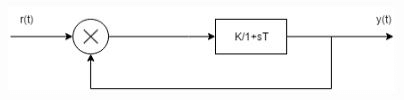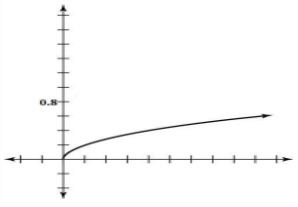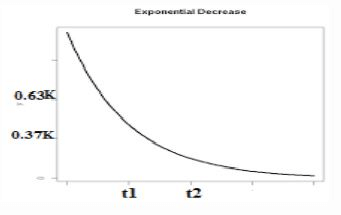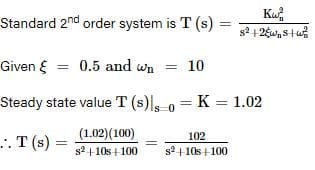# Test: Time Response Of First Order Systems

## 10 Questions MCQ Test | Test: Time Response Of First Order Systems

Description
Attempt Test: Time Response Of First Order Systems | 10 questions in 10 minutes | Mock test for Electrical Engineering (EE) preparation | Free important questions MCQ to study for Electrical Engineering (EE) Exam | Download free PDF with solutions
QUESTION: 1

### First order system is defined as :

Solution:

Explanation: First order system is defined by total number of poles and also which is same as the order of differential equation.

QUESTION: 2

### A unit step is applied at t=0 to a first order system without time delay. The response has the value of 1.264 units at t=10 mins, and 2 units at steady state. The transfer function of the system is_____________

Solution:

Explanation: a(t)= k[1-e^-t/T] K=2
0.632= 1-e^-10/T
T=600 sec
G(s)=2/(1+600s).

QUESTION: 3

### The transfer function of the system is G(s) =100/(s+1) (s+100). For a unit step input to the system the approximate settling time for 2% criterion is:

Solution:

Explanation: G(s) =100/(s+1) (s+100)
Taking the dominant pole consideration,
S=-100 pole is not taken.
G(s)= 100/s+1
Now it is first order system, ts=4T=4 sec.

QUESTION: 4

If a first order system and its time response to a unit step are as shown below, the gain K is :Solution:

Explanation: Y(s)/R(s)=K/1+sT+K
By, use of partial fraction, K/T/s+(K+1/T)
Taking inverse Laplace transform on both the sides
Y(t)=K/K+1[1-e^(K+1/T)t] K=4

QUESTION: 5

The unit impulse response of a system having transfer function K/(s+a) is shown below. The value of a is :Solution:

Explanation: G(s) = K/s+a
C(s) =K/(s+a) Since R(s) =1
C(t) =Ke^-at
T=1/a
C(t) =0.37K
T= t2=1/a.

QUESTION: 6

A system with transfer function 1/Ts+1, subjected to a step input takes to seconds to reach 50% of step height. The value of t is :

Solution:

Explanation: The response of a first order system is:
A(t)=a[1-e^-t/T] ½= 1-e^-10/t
T= 14.43 sec.

QUESTION: 7

A second-order real system has the following properties:

The damping ratio ξ=0.5ξ=0.5 and undamped natural frequency ωn=10rad/sωn=10rad/s, the steady state value at zero is 1.02.

The transfer function of the system is

Solution:QUESTION: 8

Assertion (A): It is observed that step function is first derivative of a ramp function and impulse function is first derivative of a step function.
Reason (R): From the derived time response expression it is concluded that the output time response also follows the same sequence as that of input functions.

Solution:

Explanation: If response due to one standard signal is known then response due to other signals can also be derived.

QUESTION: 9

Laplace transform of unit impulse signal is :

Solution:Use Code STAYHOME200 and get INR 200 additional OFF Use Coupon Code